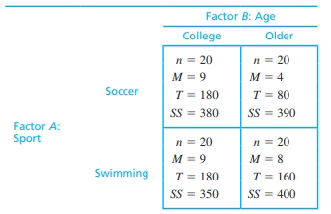Chapter 13, Problem 4REEssentials of Statistics for the B...

8th Edition
Frederick J Gravetter + 1 other
ISBN: 9781133956570

Solutions

Chapter
SectionEssentials of Statistics for the B...

8th Edition
Frederick J Gravetter + 1 other
ISBN: 9781133956570
Textbook Problem

Most sports injuries are immediate and obvious, like a broken leg. However, some can be more subtle, like the neurological damage that may occur when soccer players repeatedly head a soccer ball. To examine long-term effects of repeated heading. Downs and Abwender (2002) examined two different age groups of soccer players and swimmers. The dependent variable was performance on a conceptual thinking task. Following are hypothetical data, similar to the research results. a. Use a two-factor ANOVA with α= .05 to evaluate the main effects and interaction. b. Calculate the effects size (η2) for the main effects and the interaction. c. Briefly describe the outcome of the study.ΣX2 = 6360

a.

To determine

To test: Whether there is any significant difference between the treatment means.

To test: Whether the interaction effect exists between the two factors.

Explanation

Given info:

The data represents the Downs and Abwender of two different age groups of soccer players and swimmers with the dependent variable performance on a conceptual thinking task. The level of significance is α=0.05.

Calculation:

The test hypotheses for main effects of sports are given below:

Null hypothesis:

H0:μ1=μ2=μ3

Alternate hypothesis:

Ha:Atleast one treatment mean of sports significantly differs from the other

The test hypotheses for main effects of age are given below:

Null hypothesis:

H0:μ1=μ2=μ3

Alternate hypothesis:

Ha:Atleast one treatment mean of age significantly differs from the other

The test hypotheses for interaction effects are given below:

Null hypothesis:

H0:The interaction effect does not exists between the two factors

Alternate hypothesis:

Ha:The interaction effect exists between the two factors

Degrees of freedom for within group effects:

dfwithin=19+19+19+19=76

Total degrees of freedom:

dftotal=N1=79

Degrees of freedom for between group effects:

dfbetween=number_of_treatment1=41=3

Sum of squares:

The sum of squares for treatment A is,

SSA=T2rownrowG2N=260240+340240600280=80

Thus, the sum of squares for treatment A is SSA=80

The sum of squares for treatment B is,

SSB=T2columnncolumnG2N=360240+240240+600280180260=180

Thus, the sum of squares for treatment B is SSB=180

The sum of squares for interaction of treatment A and treatment B is,

SA×B=SSbetween_treatmentSSASSB=34080180=80

Thus, the interaction sum of squares is SA×B=80

The sum of squares of total is,

SStotal=X2G2N=6360600280=1860

Thus, thetotal sum of squares is SStotal=1,860

The treatment within sum of squares is,

SSwithin=SSINSIDE=380+390+350+400=1520

Thus, the treatment within sum of squares is SS

b.

To determine

To Explain: The results of ANOVA

Still sussing out bartleby?

Check out a sample textbook solution.

See a sample solution

The Solution to Your Study Problems

Bartleby provides explanations to thousands of textbook problems written by our experts, many with advanced degrees!

Get Started

Evaluate the integral. /6/2csctcottdt

Single Variable Calculus: Early Transcendentals, Volume I

True or False: Let . Then f(x, y) is a joint probability function.

Study Guide for Stewart's Multivariable Calculus, 8th

limx6(x210x)=. a) 24 b) 6 c) 26 d) does not exist

Study Guide for Stewart's Single Variable Calculus: Early Transcendentals, 8th